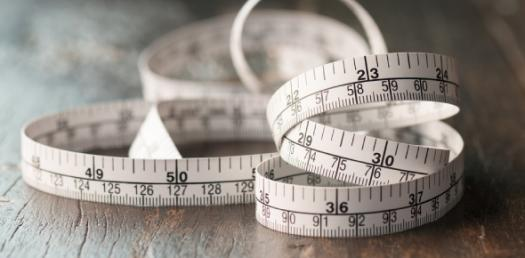# Measures Of Central Tendency And Dispersion

100 Questions | Attempts: 1979
ShareSettings• 1.
Measures of central tendency for a given set of observations measures
• A.

The scatterness of the observations

• B.

The central location of the observations

• C.

Both (i) and (ii)

• D.

None of these.

• 2.
While computing the AM from a grouped frequency distribution, we assume that
• A.

The classes are of equal length

• B.

The classes have equal frequency

• C.

All the values of a class are equal to the mid-value of that class

• D.

None of these.

• 3.
Which of the following statements is wrong?
• A.

Mean is rigidly defined

• B.

Mean is not affected due to sampling fluctuations Ensues

• C.

Mean has some mathematical properties

• D.

All these

• 4.
Which of the following statements is true?
• A.

Usually mean is the best measure of central tendency

• B.

Usually median is the best measure'of central tendency

• C.

Usually mode is the best measure of central tendency

• D.

Normally, GM is the best measure of central tendency

• 5.
For open-end classification, which of the following is the best measure of central tendency?
• A.

AM

• B.

GM

• C.

Median

• D.

Mode

• 6.
The presence of extreme observations does not affect
• A.

AM

• B.

Median

• C.

Mode

• D.

Any of these.

• 7.
In case of an even number of observations which of the following is median ?
• A.

Any of the two middle-most value

• B.

The simple average of these two middle values

• C.

The weighted average of these two middle values

• D.

Any of these

• 8.
The most commonly used measure of central tendency is
• A.

AM

• B.

Median

• C.

Mode

• D.

Both GM and HM

• 9.
Which one of the following is not uniquely defined?
• A.

Mean

• B.

Median

• C.

Mode

• D.

All of these measures

• 10.
Which of the following measure of the central tendency is difficult to compute?
• A.

Mean

• B.

Median

• C.

Mode

• D.

Gm

• 11.
Which measure(s) of central tendency is(are) considered for finding the average rates:
• A.

AM

• B.

GM

• C.

HM

• D.

Both (ii)and(iii)

• 12.
For a moderately skewed distribution, which of he following relationship holds?
• A.

Mean - Mode = 3 (Mean - Median)

• B.

Median - Mode = 3 (Mean - Median)

• C.

Mean - Median = 3 (Mean - Mode)

• D.

Mean - Median = 3 (Median - Mode)

• 13.
Weighted averages are considered when
• A.

The data are not classified

• B.

The data are put in the form of grouped frequency distribution

• C.

All the observations are not of equal importance

• D.

Both (i) and (iii).

• 14.
Which of the following results hold for a set of distinct positive observations?
• A.

AM >= GM >= HM

• B.

HM >= GM >= AM

• C.

AM > GM > HM

• D.

GM > AM > HM

• 15.
When a firm registers both profits and losses, which of the following measure of central tendency cannot be considered?
• A.

AM

• B.

GM

• C.

Median

• D.

Mode

• 16.
Quartiles are the values dividing a given set of observations into
• A.

Two equal parts

• B.

Four equal parts

• C.

Five equal parts

• D.

None of these

• 17.
Quartiles can be determined graphically using
• A.

Histogram

• B.

Frequency Polygon

• C.

Ogive

• D.

Pie chart

• 18.
Which of the following measure(s) possesses (possess) mathematical properties?
• A.

AM

• B.

GM

• C.

HM

• D.

All of these

• 19.
Which of the following measure(s) satisfies (satisfy) a linear relationship between two variables?
• A.

Mean

• B.

Median

• C.

Mode

• D.

All of these

• 20.
Which of he following measures of central tendency is based on only fifty percent of the central values?
• A.

Mean

• B.

Median

• C.

Mode

• D.

Both (i) and(ii)

• 21.
If there are 3 observations 15, 20, 25 then the sum of deviation of the observations from their AM is
• A.

0

• B.

5

• C.

-5

• D.

None of these

• 22.
What is the median for the following observations? 5, 8, 6, 9, 11, 4.
• A.

6

• B.

7

• C.

8

• D.

None of these

• 23.
What is the modal value for the numbers 5, 8, 6, 4, 10, 15, 18, 10?
• A.

18

• B.

10

• C.

14

• D.

None of these

• 24.
What is the GM for the numbers 8, 24 and 40? Options : A. 24   B. 12                         C.                     D. 10
• A.

A

• B.

B

• C.

C

• D.

D

• 25.
The harmonic mean for the numbers 2, 3, 5 is Options : A. 2.00 B. 3.33                      C. 2.90 D.
• A.

A

• B.

B

• C.

C

• D.

D

## Related TopicsBack to top
×

Wait!
Here's an interesting quiz for you.